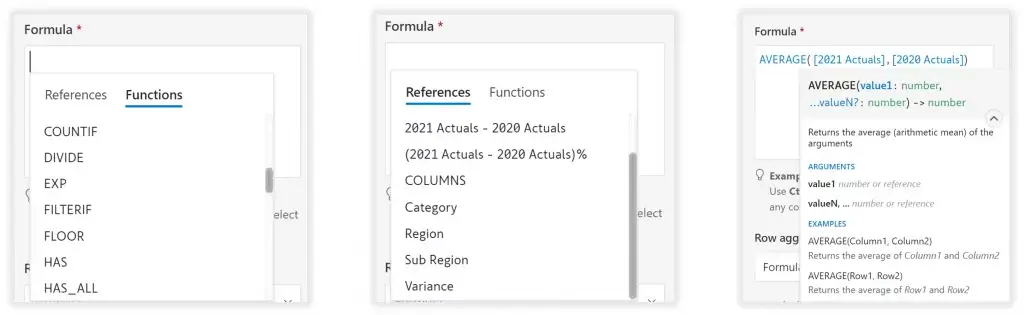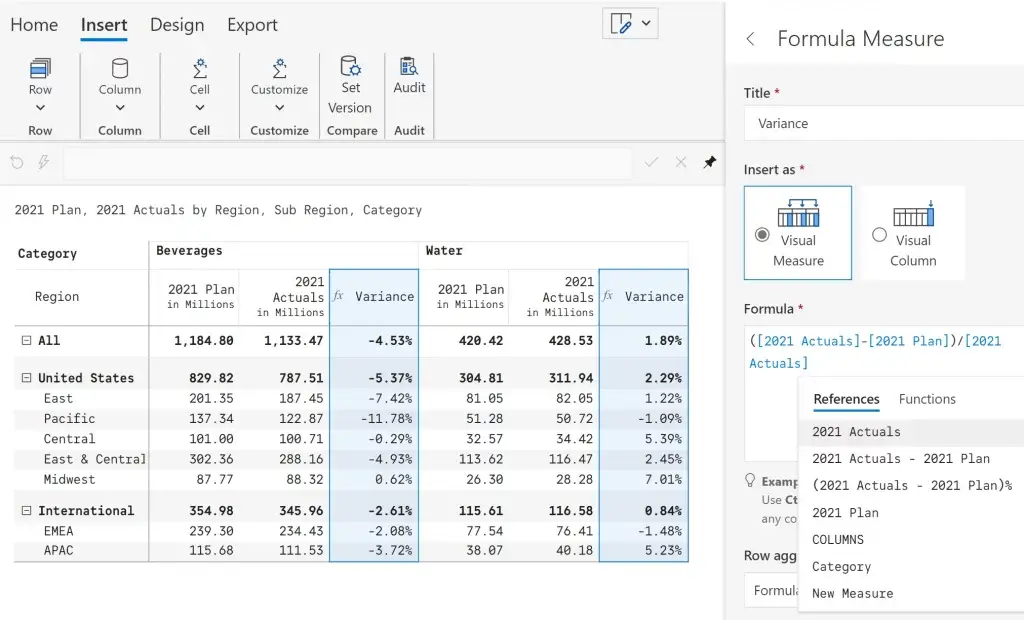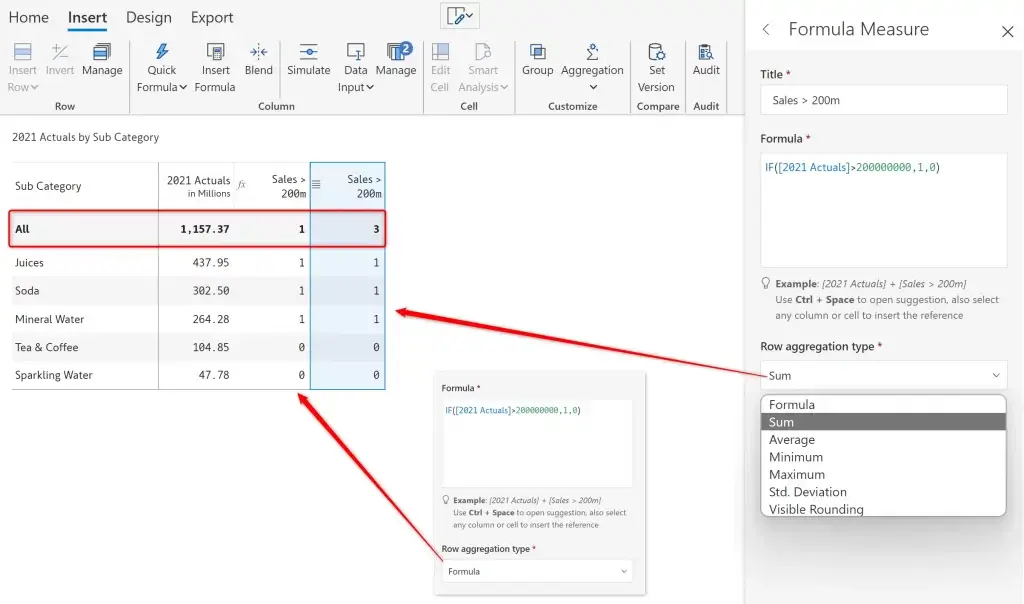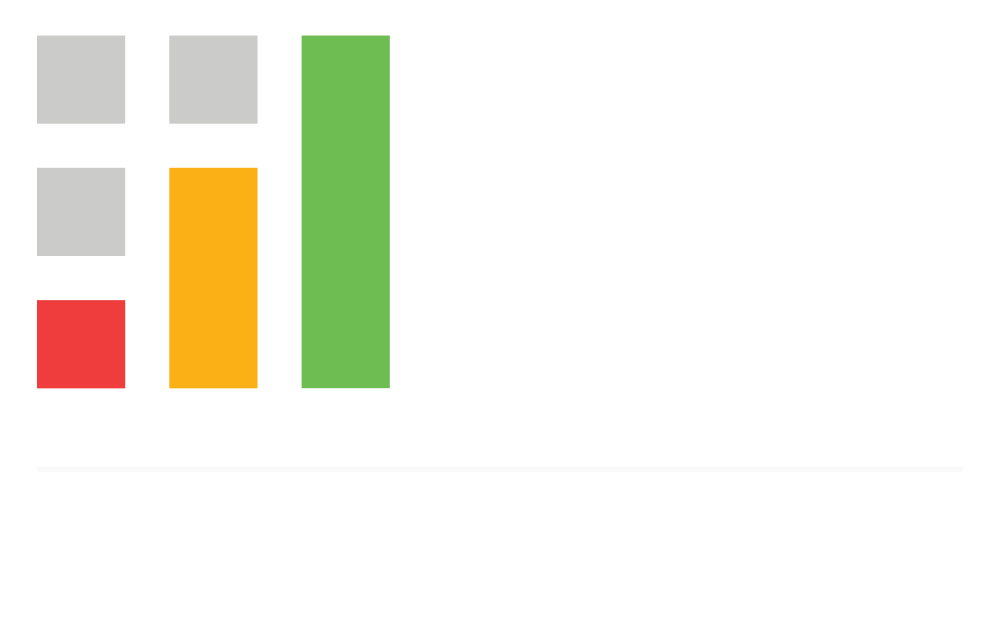Upcoming webinar on 'Inforiver Charts : The fastest way to deliver stories in Power BI', Aug 29th , Monday, 10.30 AM CST.    Register Now

# The fastest way to create visual calculationsin Power BI#### Flexible formula editor

Define visual-level formulae using logical, math, text functions and more

#### Cell-level formulae

Use spreadsheet-like formula bar to edit cell value or define formula; supports formula references to other cells

#### Insert rows, columns & measures

Manually type in values or calculate using the formula editor

#### Quick formula options

One-click options to insert columns such as % contribution to grand total, running total, ranking within group

#### Custom total aggregations

Define aggregations for totals and subtotals at the visual-level without using DAX

#### Conditional functions

Leverage functions such as if, else, switch to define conditional statements

#### Boolean functions

Define boolean expressions using functions such as and, or, xor, and not

Shift any trend data by 'n' periods using lead/lag functions

#### String functions

Manipulate text using string functions such as concatenate, left, match

## Highlights

### 1. Flexible formula editor

Leverage no-code visual formula engine which supports 50+ functions (logical, math, boolean etc.,) and identifiers. The formula editor provides syntax, examples and features such as autocomplete, multi-line support and more to help users create, and troubleshoot formulas### 2. Cell-level formulae

Use spreadsheet-like formula bar to edit cell value or formula; supports formula references to other cells### 3. Insert rows, columns & measures​

Insert static rows and columns where you can manually type in values (supports scaled entries) or define calculations using the formula editor. Calculations can also be inserted for every column hierarchy using visual measures### 4. Quick formula options​

Inforiver provides one-click options to insert columns and measures such as % contribution to grand total, running total, ranking within group. % contribution and variance columns can also be inserted based on a cell selection### 5. Custom total aggregation​

Define aggregations for totals and subtotals at the visual-level without using DAX. Inforiver provides aggregation types such as sum, average, minimum, maximum, average, visible rounding etc. out-of-the-box### 6. Conditional functions

Leverage functions such as IF, ELSE, SWITCH to define conditional statements### 7. Boolean functions

Define boolean expressions using functions such as AND, OR, XOR, and NOTShift any trend data by 'n' periods using lead/lag functions and quickly perform variance calculations such as QoQ growth, YoY growth etc### 9. String functions

Manipulate text using string functions such as CONCATENATE, LEFT, MATCH ​## Looking for more?

Explore how you can tell compelling data stories